• BTC 2.48%

\$58,866.0

• ETH 11.27%

\$3,876.78

• XRP -1.41%

\$1.56000

• XZC 0.95%

\$19.0200

• MAS %

\$0.00000

• MCASH 12.38%

\$0.00040

••••••••# Genericity of VHDL descriptions

• a multi-function operator, structurally described from an elementary cell
• a FIFO that allows the communication of messages between two asynchronous processors.

### 1. Arithmetic operator

We want to implement an arithmetic operator with the following structure: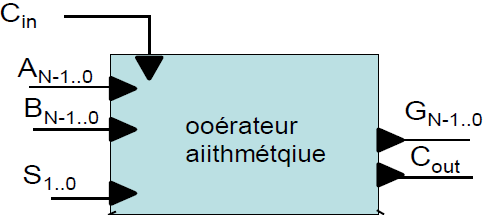We want this operator to be able to perform the following main operations:

• the transfer of the operand A
• the increment
• the addition of the two operands
• the addition of the operand A with the complement to 1 from B
• the subtraction B from A
• the decrementation of A by 1.

This has been represented according to the following functional table: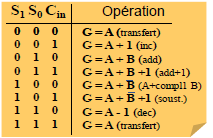We describe the elementary cell of the arithmetic operator from an entity-architecture pair as follows: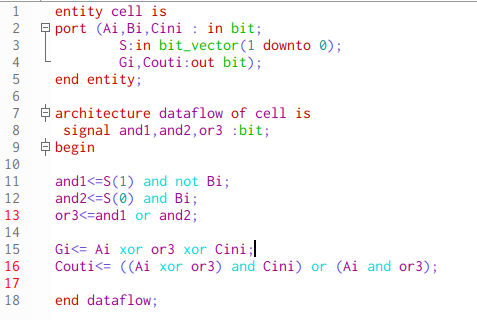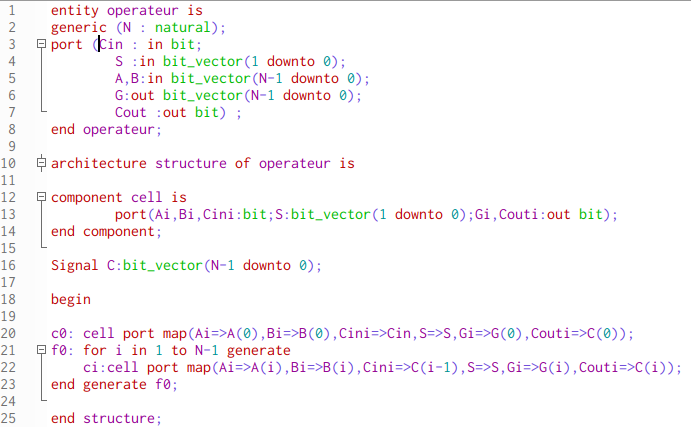We describe the following test bench to allow us to validate our operator on the operations stated previously.

We obtain the following simulation: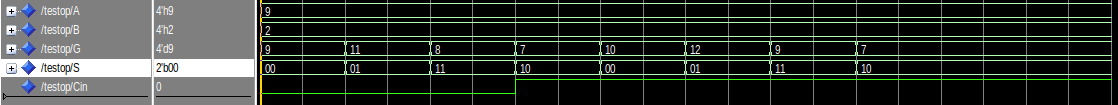The result displayed on G corresponds well to our previous functional table.

### 2. The FIFO component

We wish to describe a data storage circuit of FIFO (First in, First out) type represented as follows: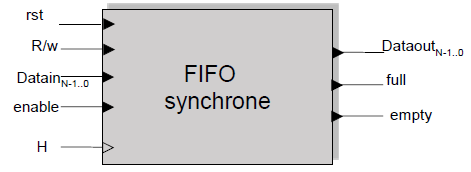• Datain: data entry to write to the FIFO
• Dataout: value of the oldest unread value in the queue
• R / W: represents the type of operation to be performed on the queue
• enable: to authorize the operation
• full: status indicator indicating whether the queue is full
• empty: status indicator indicating whether the queue is empty

We thus seek to describe the following block diagram: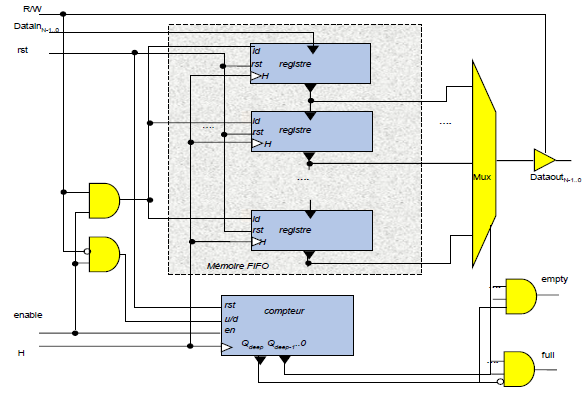We define the previous entity/architecture pair making it possible to describe the FIFO using concurrent instructions. We obtain the following chronogram: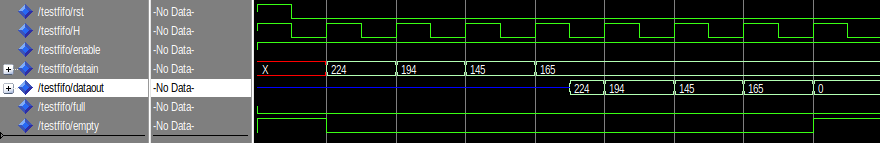`This article is part of my Electronics posts blog, in which you can find few articles about things i learnt in my post-graduated school :)`